### BAYES Statement

BAYES <options> ;

The BAYES statement requests a Bayesian analysis of the regression model by using Gibbs sampling. The Bayesian posterior samples (also known as the chain) for the regression parameters are not tabulated. The Bayesian posterior samples (also known as the chain) for the model parameters can be output to a SAS data set.

Table 51.2 summarizes the options available in the BAYES statement.

Table 51.2: BAYES Statement Options

Option

Description

Monte Carlo Options

Specifies initial values of the chain

Specifies that maximum likelihood estimates be used as initial values of the chain

Specifies the use of a Metropolis step

Specifies the number of burn-in iterations

Specifies the number of iterations after burn-in

Specifies the random number generator seed

Controls the thinning of the Markov chain

Model and Prior Options

Specifies the prior of the regression coefficients

Specifies the prior of the exponential scale parameter

Specifies the prior of the three-parameter gamma shape parameter

Specifies the prior of the scale parameter

Specifies the prior of the Weibull scale parameter

Specifies the prior of the Weibull shape parameter

Summary Statistics and Convergence Diagnostics

Displays convergence diagnostics

Displays diagnostic plots

Displays summary statistics of the posterior samples

Posterior Samples

Names a SAS data set for the posterior samples

The following list describes these options and their suboptions.

COEFFPRIOR=UNIFORM | NORMAL <(normal-options)>
CPRIOR=UNIFORM | NORMAL <(option)>
COEFF=UNIFORM | NORMAL <(option)>

specifies the prior distribution for the regression coefficients. The default is COEFFPRIOR=UNIFORM. The available prior distributions are as follows:

NORMAL<(normal-option)>

specifies a normal distribution. The normal-options include the following:

CONDITIONAL

specifies that the normal prior, conditional on the current Markov chain value of the location-scale model precision parameter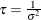, is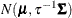, where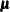and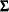are the mean and covariance of the normal prior specified by other normal options.

INPUT= SAS-data-set

specifies a SAS data set that contains the mean and covariance information of the normal prior. The data set must have a _TYPE_ variable to represent the type of each observation and a variable for each regression coefficient. If the data set also contains a _NAME_ variable, the values of this variable are used to identify the covariances for the _TYPE_=’COV’ observations; otherwise, the _TYPE_=’COV’ observations are assumed to be in the same order as the explanatory variables in the MODEL statement. PROC LIFEREG reads the mean vector from the observation with _TYPE_=’MEAN’ and reads the covariance matrix from observations with _TYPE_=’COV’. For an independent normal prior, the variances can be specified with _TYPE_=’VAR’; alternatively, the precisions (inverse of the variances) can be specified with _TYPE_=’PRECISION’.

RELVAR<=c>

specifies the normal prior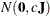, where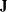is a diagonal matrix with diagonal elements equal to the variances of the corresponding ML estimator. By default,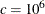.

VAR<=c>

specifies the normal prior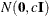, whereis the identity matrix.

If you do not specify an option, the normal prior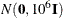, whereis the identity matrix, is used. See the section Normal Prior for more details.

UNIFORM

specifies a flat prior—that is, the prior that is proportional to a constant (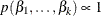for all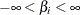).

DIAGNOSTICS=ALL | NONE | (keyword-list)
DIAG=ALL | NONE | (keyword-list)

controls the number of diagnostics produced. You can request all the following diagnostics by specifying DIAGNOSTICS=ALL. If you do not want any of these diagnostics, specify DIAGNOSTICS=NONE. If you want some but not all of the diagnostics, or if you want to change certain settings of these diagnostics, specify a subset of the following keywords. The default is DIAGNOSTICS=(AUTOCORR ESS GEWEKE).

AUTOCORR <(LAGS= numeric-list)>

computes the autocorrelations of lags given by LAGS= list for each parameter. Elements in the list are truncated to integers and repeated values are removed. If the LAGS= option is not specified, autocorrelations of lags 1, 5, 10, and 50 are computed for each variable. See the section Autocorrelations for details.

ESS

computes Carlin’s estimate of the effective sample size, the correlation time, and the efficiency of the chain for each parameter. See the section Effective Sample Size for details.

GELMAN <(gelman-options)>

computes the Gelman and Rubin convergence diagnostics. You can specify one or more of the following gelman-options:

NCHAIN=number
N=number

specifies the number of parallel chains used to compute the diagnostic, and must be 2 or larger. The default is NCHAIN=3. If an INITIAL= data set is used, NCHAIN defaults to the number of rows in the INITIAL= data set. If any number other than this is specified with the NCHAIN= option, the NCHAIN= value is ignored.

ALPHA=value

specifies the significance level for the upper bound. The default is ALPHA=0.05, resulting in a 97.5% bound.

See the section Gelman and Rubin Diagnostics for details.

GEWEKE <(geweke-options)>

computes the Geweke spectral density diagnostics, which are essentially a two-sample t test between the first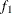portion and the last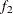portion of the chain. The default is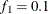and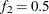, but you can choose other fractions by using the following geweke-options:

FRAC1=value

specifies the fractionfor the first window.

FRAC2=value

specifies the fractionfor the second window.

See the section Geweke Diagnostics for details.

HEIDELBERGER <(heidel-options)>

computes the Heidelberger and Welch diagnostic for each variable, which consists of a stationarity test of the null hypothesis that the sample values form a stationary process. If the stationarity test is not rejected, a halfwidth test is then carried out. Optionally, you can specify one or more of the following heidel-options:

SALPHA=value

specifies the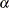level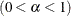for the stationarity test.

HALPHA=value

specifies thelevelfor the halfwidth test.

EPS=value

specifies a positive numbersuch that if the halfwidth is less thantimes the sample mean of the retained iterates, the halfwidth test is passed.

See the section Heidelberger and Welch Diagnostics for details.

MCSE
MCERROR

computes the Monte Carlo standard error for each parameter. The Monte Caro standard error, which measures the simulation accuracy, is the standard error of the posterior mean estimate and is calculated as the posterior standard deviation divided by the square root of the effective sample size. See the section Standard Error of the Mean Estimate for details.

RAFTERY<(raftery-options)>

computes the Raftery and Lewis diagnostics that evaluate the accuracy of the estimated quantile (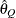for a given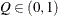) of a chain.can achieve any degree of accuracy when the chain is allowed to run for a long time. A stopping criterion is when the estimated probability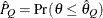reaches within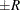of the value Q with probability S; that is,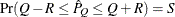. The following raftery-options enable you to specify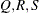, and a precision levelfor the test:

QUANTILE | Q=value

specifies the order (a value between 0 and 1) of the quantile of interest. The default is 0.025.

ACCURACY | R=value

specifies a small positive number as the margin of error for measuring the accuracy of estimation of the quantile. The default is 0.005.

PROBABILITY | S=value

specifies the probability of attaining the accuracy of the estimation of the quantile. The default is 0.95.

EPSILON | EPS=value

specifies the tolerance level (a small positive number) for the stationary test. The default is 0.001.

See the section Raftery and Lewis Diagnostics for details.

EXPSCALEPRIOR=GAMMA<(options)> | IMPROPER
ESCALEPRIOR=GAMMA<(options)> | IMPROPER
ESCPRIOR=GAMMA<(options)> | IMPROPER

specifies that Gibbs sampling be performed on the exponential distribution scale parameter and the prior distribution for the scale parameter. This prior distribution applies only when the exponential distribution and no covariates are specified.

A gamma prior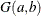with density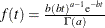is specified by EXPSCALEPRIOR=GAMMA, which can be followed by one of the following gamma-options enclosed in parentheses. The hyperparameters a and b are the shape and inverse-scale parameters of the gamma distribution, respectively. See the section Gamma Prior for more details. The default is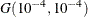.

RELSHAPE<=c>

specifies independent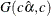distribution, where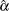is the MLE of the exponential scale parameter. With this choice of hyperparameters, the mean of the prior distribution isand the variance is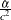. By default, c=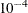.

SHAPE=a
ISCALE=b

when both specified, results in aprior.

SHAPE=c

when specified alone, results in a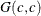prior.

ISCALE=c

when specified alone, results in aprior.

An improper prior with density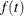proportional to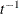is specified with EXPSCALEPRIOR=IMPROPER.

GAMMASHAPEPRIOR=NORMAL<(options)>
GAMASHAPEPRIOR=NORMAL<(options)>
SHAPE1PRIOR=NORMAL<(options)>

specifies the prior distribution for the gamma distribution shape parameter. If you do not specify any options in a gamma model, the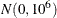prior for the shape is used. You can specify MEAN= and VAR= or RELVAR= options, either alone or together, to specify the mean and variance of the normal prior for the gamma shape parameter.

MEAN=a

specifies a normal prior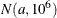. By default, a=0.

RELVAR<=b>

specifies the normal prior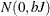, where J is the variance of the MLE of the shape parameter. By default, b=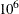.

VAR=c

specifies the normal prior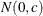. By default, c=.

INITIAL=SAS-data-set

specifies the SAS data set that contains the initial values of the Markov chains. The INITIAL= data set must contain all the variables of the model. You can specify multiple rows as the initial values of the parallel chains for the Gelman-Rubin statistics, but posterior summaries, diagnostics, and plots are computed only for the first chain. If the data set also contains the variable _SEED_, the value of the _SEED_ variable is used as the seed of the random number generator for the corresponding chain.

INITIALMLE

specifies that maximum likelihood estimates of the model parameters be used as initial values of the Markov chain. If this option is not specified, estimates of the mode of the posterior distribution obtained by optimization are used as initial values.

METROPOLIS=YES
METROPOLIS=NO

specifies the use of a Metropolis step to generate Gibbs samples for posterior distributions that are not log concave. The default value is METROPOLIS=YES.

NBI=number

specifies the number of burn-in iterations before the chains are saved. The default is 2000.

NMC=number

specifies the number of iterations after the burn-in. The default is 10000.

OUTPOST=SAS-data-set
OUT=SAS-data-set

names the SAS data set that contains the posterior samples. See the section OUTPOST= Output Data Set for more information. Alternatively, you can create the output data set by specifying an ODS OUTPUT statement as follows:

ODS OUTPUT POSTERIORSAMPLE=SAS-data-set

PLOTS<(global-plot-options)>= plot-request
PLOTS<(global-plot-options)>= (plot-request < …plot-request>)

controls the display of diagnostic plots. Three types of plots can be requested: trace plots, autocorrelation function plots, and kernel density plots. By default, the plots are displayed in panels unless the global plot option UNPACK is specified. Also, when specifying more than one type of plots, the plots are displayed by parameters unless the global plot option GROUPBY is specified. When you specify only one plot request, you can omit the parentheses around the plot request. For example:

 plots=none
plots(unpack)=trace
plots=(trace autocorr)


ODS Graphics must be enabled before plots can be requested. For example:

ods graphics on;
proc lifereg;
model y=x;
bayes plots=trace;
run;
ods graphics off;


For more information about enabling and disabling ODS Graphics, see the section Enabling and Disabling ODS Graphics in Chapter 21: Statistical Graphics Using ODS.

The global plot options are as follows:

FRINGE

creates a fringe plot on the X axis of the density plot.

GROUPBY=PARAMETER
GROUPBY=TYPE

specifies how the plots are grouped when there is more than one type of plot.

GROUPBY=TYPE

specifies that the plots be grouped by type.

GROUPBY=PARAMETER

specifies that the plots be grouped by parameter.

GROUPBY=PARAMETER is the default.

LAGS=n

specifies that autocorrelations be plotted up to lag n. If this option is not specified, autocorrelations are plotted up to lag 50.

SMOOTH

displays a fitted penalized B-spline curve for each trace plot.

UNPACKPANEL
UNPACK

specifies that all paneled plots be unpacked, meaning that each plot in a panel is displayed separately.

The plot requests include the following:

ALL

specifies all types of plots. PLOTS=ALL is equivalent to specifying PLOTS=(TRACE AUTOCORR DENSITY).

AUTOCORR

displays the autocorrelation function plots for the parameters.

DENSITY

displays the kernel density plots for the parameters.

NONE

suppresses all diagnostic plots.

TRACE

displays the trace plots for the parameters. See the section Visual Analysis via Trace Plots for details.

SCALEPRIOR=GAMMA<(options)>

specifies that Gibbs sampling be performed on the location-scale model scale parameter and the prior distribution for the scale parameter.

A gamma priorwith densityis specified by SCALEPRIOR=GAMMA, which can be followed by one of the following gamma-options enclosed in parentheses. The hyperparameters a and b are the shape and inverse-scale parameters of the gamma distribution, respectively. See the section Gamma Prior for details. The default is.

RELSHAPE<=c>

specifies independent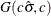distribution, where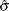is the MLE of the scale parameter. With this choice of hyperparameters, the mean of the prior distribution isand the variance is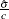. By default, c=.

SHAPE=a
ISCALE=b

when both specified, results in aprior.

SHAPE=c

when specified alone, results in aprior.

ISCALE=c

when specified alone, results in aprior.

SEED=number

specifies an integer seed in the range 1 to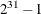for the random number generator in the simulation. Specifying a seed enables you to reproduce identical Markov chains for the same specification. If the SEED= option is not specified, or if you specify a nonpositive seed, a random seed is derived from the time of day.

STATISTICS <(global-options)> = ALL | NONE | keyword | (keyword-list)
STATS <(global-statoptions)> = ALL | NONE | keyword | (keyword-list)

controls the number of posterior statistics produced. Specifying STATISTICS=ALL is equivalent to specifying STATISTICS= (SUMMARY INTERVAL COV CORR). If you do not want any posterior statistics, you specify STATISTICS=NONE. The default is STATISTICS=(SUMMARY INTERVAL). See the section Summary Statistics for details. The global-options include the following:

ALPHA=numeric-list

controls the probabilities of the credible intervals. The ALPHA= values must be between 0 and 1. Each ALPHA= value produces a pair of 100(1–ALPHA)% equal-tail and HPD intervals for each parameters. The default is ALPHA=0.05, which yields the 95% credible intervals for each parameter.

PERCENT=numeric-list

requests the percentile points of the posterior samples. The PERCENT= values must be between 0 and 100. The default is PERCENT=25, 50, 75, which yields the 25th, 50th, and 75th percentile points, respectively, for each parameter.

The list of keywords includes the following:

CORR

produces the posterior correlation matrix.

COV

produces the posterior covariance matrix.

SUMMARY

produces the means, standard deviations, and percentile points for the posterior samples. The default is to produce the 25th, 50th, and 75th percentile points, but you can use the global PERCENT= option to request specific percentile points.

INTERVAL

produces equal-tail credible intervals and HPD intervals. The default is to produce the 95% equal-tail credible intervals and 95% HPD intervals, but you can use the global ALPHA= option to request intervals of any probabilities.

NONE

suppresses printing all summary statistics.

THINNING=number
THIN=number

controls the thinning of the Markov chain. Only one in every k samples is used when THINNING=k, and if NBI=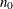and NMC=n, the number of samples kept is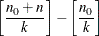where [a] represents the integer part of the number a. The default is THINNING=1.

WEIBULLSCALEPRIOR=GAMMA<(options)>
WSCALEPRIOR=GAMMA<(options)>
WSCPRIOR=GAMMA<(options)>

specifies that Gibbs sampling be performed on the Weibull model scale parameter and the prior distribution for the scale parameter. This option applies only when a Weibull distribution and no covariates are specified. When this option is specified, PROC LIFEREG performs Gibbs sampling on the Weibull scale parameter, which is defined as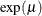, whereis the intercept term.

A gamma prioris specified by WEIBULLSCALEPRIOR=GAMMA, which can be followed by one of the following gamma-options enclosed in parentheses. The gamma probability density is given by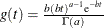. The hyperparameters a and b are the shape and inverse-scale parameters of the gamma distribution, respectively. See the section Gamma Prior for details about the gamma prior. The default is.

RELSHAPE<=c>

specifies independentdistribution, whereis the MLE of the Weibull scale parameter. With this choice of hyperparameters, the mean of the prior distribution isand the variance is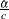. By default, c=.

SHAPE=a
ISCALE=b

when both specified, results in aprior.

SHAPE=c

when specified alone, results in aprior.

ISCALE=c

when specified alone, results in aprior.

WEIBULLSHAPEPRIOR=GAMMA<(options)>
WSHAPEPRIOR=GAMMA<(options)>
WSHPRIOR=GAMMA<(options)>

specifies that Gibbs sampling be performed on the Weibull model shape parameter and the prior distribution for the shape parameter. When this option is specified, PROC LIFEREG performs Gibbs sampling on the Weibull shape parameter, which is defined as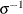, where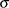is the location-scale model scale parameter.

A gamma priorwith densityis specified by WEIBULLSHAPEPRIOR=GAMMA, which can be followed by one of the following gamma-options enclosed in parentheses. The hyperparameters a and b are the shape and inverse-scale parameters of the gamma distribution, respectively. See the section Gamma Prior for details about the gamma prior. The default is.

RELSHAPE<=c>

specifies independent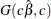distribution, where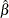is the MLE of the Weibull shape parameter. With this choice of hyperparameters, the mean of the prior distribution isand the variance is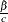. By default, c=.

SHAPE<=a>
ISCALE=b

when both specified, results in aprior.

SHAPE=c

when specified alone, results in aprior.

ISCALE=c

when specified alone, results in aprior.